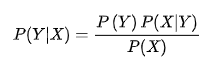# 朴素贝叶斯法（对于连续和离散属性的处理）

+关注继续查看

## 1.贝叶斯分类模型

贝叶斯分类模型如下：其中，X表示属性集，Y表示类变量，P(Y)为先验概率，P(X|Y)为类条件概率，P(X)为证据，P(Y|X)为后验概率。贝叶斯分类模型就是用先验概率P(Y)、类条件概率P(X|Y)和证据P(X)来表示后验概率。在比较Y的后验概率时，分母中的证据P(X)总是常数，因此可以忽略不计。先验概率P(Y)可以通过计算训练集中属于每个类的训练记录所占的比例很容易估计。对类条件概率P(X|Y)的估计，不同的实现决定不同的贝叶斯分类方法，常见的有朴素贝叶斯分类法和贝叶斯信念网络。

## 2.朴素贝叶斯分类模型## 3.实例

数据集如下：从该数据集计算得到的先验概率以及每个离散属性的类条件概率、连续属性的类条件概率分布的参数（样本均值和方差）如下：

P(有房=是|No) = 3/7

P(有房=否|No) = 4/7

P(有房=是|Yes) = 0

P(有房=否|Yes) = 1

P(婚姻状况=单身|No) = 2/7

P(婚姻状况=离婚|No) = 1/7

P(婚姻状况=已婚|No) = 4/7

P(婚姻状况=单身|Yes) = 2/3

P(婚姻状况=离婚|Yes) = 1/3

P(婚姻状况=已婚|Yes) = 0

——》待预测记录：X={有房=否，婚姻状况=已婚，年收入=120K}

P(No)*P(有房=否|No)*P(婚姻状况=已婚|No)*P(年收入=120K|No)=0.7*4/7*4/7*0.0072=0.0024

P(Yes)*P(有房=否|Yes)*P(婚姻状况=已婚|Yes)*P(年收入=120K|Yes)=0.3*1*0*1.2*10-9=0http://blog.csdn.net/yan456jie/article/details/5264978829 014 0Part3__机器学习实战学习笔记__朴素贝叶斯

87 0993 04578 01342 01055 01674 0def loadDataSet(): postingList=[['my', 'dog', 'has', 'flea', 'problems', 'help', 'please'], ['m...
829 0
36

0